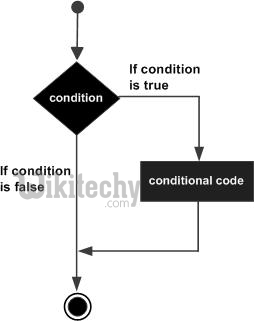# java tutorial - Java Operator if - java programming - learn java - java basics - java for beginnersLearn Java - Java tutorial - Java operator if - Java examples - Java programs

Operator if — it consists of a logical expression followed by one or more statements.

## Syntax

The syntax of the if statement in Java is:

• If the logical expression is true, the block of code within the if statement will be executed. If not, the following code block following the if statement (after the closing curly brace) will be executed.

## Process descriptionLearn java - java tutorial - if-operator - java examples - java programs

## The conditional operator if

• Creating branching in programs, making decisions, checking conditions, exceptional situations, checking for errors.
• name: if (condition) then operators_T else operators_F end if name
• name: if (condition) then operators_T end if name
• if (condition) operator_T